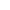Physics | ICSE Board | Grade 12 | 2013
Q. You are provided with one low resistance RL and one high resistance RH and two galvanometers. One galvanometer is to be converted to an ammeter and the other to a voltmeter. Show how you will do this with the help of simple, labelled diagrams
View SolutionQ.
(i) Write an expression (derivation not required) for intensity of electric field in:
(1) Axial position.
(2) Broad side position of an electric dipole, in terms of its length (2a) dipole moment (p) and distance (r).
(ii) What is the ratio of these two intensities i.e. E1 : E2, for a short electric dipole?
View SolutionQ. Name the series of lines in the hydrogen spectrum which lies in the ultra-violet region:
View SolutionQ. Give an example where energy is converted into matter
View SolutionQ. What is Modulation? Explain in brief
View SolutionQ. Plot a labelled graph to show variation of thermo-emf e versus temperature difference θ between the two junctions of a thermocouple. Mark N as neutral temperature and I as temperature of inversion
View SolutionQ. What is the shape of the wavefront diverging from a point source of light?
View SolutionQ. In an astronomical telescope of refracting type:
1. Eyepiece has greater focal length
2. Objective has greater focal length
3. Objective and eyepiece have equal focal length
4. Eyepiece has greater aperture than the objective
View SolutionQ. Write an expression (derivation not required) for intensity of electric field of an electric dipole, in terms of its length (2a), dipole moment (p) and distance (r) for:
(1) Axial position.
View SolutionQ. Fill in the blank in the given nuclear reaction:
__________ +2713Al2512Mg+42He
View SolutionQ. Three capacitors C1=6μF, C2=12μF and C3=20μF are connected to a 100 V battery, as shown following figure below:
Calculate:
Electrostatic potential energy stored in capacitor C3View SolutionQ. Five resistors are connected as shown in adjoining figure.
The effective resistance i.e., equivalent resistance between the points A and B is1. 5Ω
2. 15Ω
3. 20Ω
4. 4Ω
View SolutionQ. The critical angle for a given transparent medium and air is ic. A ray of light travelling in air is incident on this transparent medium at an angle of incident equal to the polarising angle ip. What is the relation between the two angles ic and ip?
View SolutionQ. n cells, each of emf e and internal resistance r are joined in series to form a row. m such rows are connected in parallel to form a battery of N=mn cells. This battery is connected to an external resistance R
Show that current I flowing through the external resistance R is given by:
I=NemR+nr
View SolutionQ. The Biot Savart's Law in vector form is
1. ¯¯¯¯¯¯¯δB=μ04πdl(l×r)r3
2. ¯¯¯¯¯¯¯δB=μ04πI(dl×r)r3
3. ¯¯¯¯¯¯¯δB=μ04πI(r×dl)r3
4. ¯¯¯¯¯¯¯δB=μ04πI(dl×r)r2
View SolutionQ. Alternating current flowing through a certain electrical device leads over the potential difference across it by 90. State whether this device is a resistor, capacitor or an inductor
View SolutionQ. Relative permittivity of water is 81. If ϵw and ϵ0 are permittivities of water and vacuum respectively, then :
1. ϵ0=9ϵw
2. ϵ0=81ϵw
3. ϵw=9ϵ0
4. ϵw=81ϵ0
View SolutionQ. In the circuit shown in figure belwo, E1=17V, E2=21 V, R1=2Ω, R23Ω and R3=5Ω. Using Kirchhoff's laws, find the currents flowing through the resistors R1, R2 and R3. (Internal resistance of each of the batteries is negligible)View SolutionQ. The particles which cannot be accelerated by a cyclotron or a Van de Graff generator are :
1. alpha particles
2. beta particles
3. neutrons
4. protons
View SolutionQ. A large hollow metallic sphere has a positive charge of 35.4μC at its centre. Find how much electric flux will emanate from the sphere ?
View SolutionQ. Find the focal length and nature of a lens whose optical power is 5D
View SolutionQ. What is Peltier effect?
View SolutionQ. What are the dark lines seen in the solar spectrum called?
View SolutionQ. State the SI unit of magnetic dipole moment
View SolutionQ. To convert a pure semiconductor into n-type semi-conductor, what type of impurity is added to it?
View SolutionQ. What is the relation between wavelength and momentum of moving particles?
View SolutionQ. Name any one instrument which works on the principle of tangent law in magnetism
View SolutionQ. A current 'I' flows through a metallic wire of radius 'r' and the free electrons in it drift with a velocity vd. Calculate the drift velocity of the free electrons through the wire of the same material, having double the radius, when some current flows through it.
View SolutionQ. Three capacitors C1=6μF, C2=12μF and C3=20μF are connected to a 100 V battery, as shown following figure below:
Calculate:
Charge on each plate of capacitor C1View SolutionQ. n cells, each of emf e and internal resistance r are joined in series to form a row. m such rows are connected in parallel to form a battery of N=mn cells. This battery is connected to an external resistance R
What is the emf of this battery and how much is its internal resistance?
View Solution